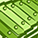QlikView App Dev

Discussion Board for collaboration related to QlikView App Development.

Announcements
QlikWorld 2022, LIVE in Denver CO., May 16-19, 2022. REGISTER NOW TO RECEIVE EARLY BIRD PRICING
cancel
Showing results for
Did you mean:Partner

Convert Nested If's to Div: Pivot Table Acting As Heatmap Background Color

Hello,

The following background color equation for my pivot table works great in the qlikview app, but when posted on the server it flashes and the pivot table object is blank white. I think I am using too many nested if statements for it to work when moved to the server.

Can anyone help me convert the below equation using the Div Function?

NESTED IFS

If(IsNull(Avg(DEMAND)), RGB(0, 0, 0),

IF(div((Avg(DEMAND)-MIN(total <Month> DEMAND)), ((MAX(total <Month> DEMAND) - MIN(total <Month> DEMAND))/12)) = 0 ,        RGB(245, 255, 240),

If(\$(vScale0) <= Avg(DEMAND) and Avg(DEMAND) < \$(vScale1), RGB(245, 255, 240),

If(\$(vScale1) <= Avg(DEMAND) and Avg(DEMAND) < \$(vScale2), RGB(193, 255, 193),

If(\$(vScale2) <= Avg(DEMAND) and Avg(DEMAND) < \$(vScale3), RGB(180, 238, 180),

If(\$(vScale3) <= Avg(DEMAND) and Avg(DEMAND) < \$(vScale4), RGB(0, 250, 154),

If(\$(vScale4) <= Avg(DEMAND) and Avg(DEMAND) < \$(vScale5), RGB(179, 238, 58),

If(\$(vScale5) <= Avg(DEMAND) and Avg(DEMAND) < \$(vScale6), RGB(192, 255, 62),

If(\$(vScale6) <= Avg(DEMAND) and Avg(DEMAND) < \$(vScale7), RGB(255, 255, 0),

If(\$(vScale7) <= Avg(DEMAND) and Avg(DEMAND) < \$(vScale8), RGB(255, 204, 0),

If(\$(vScale8) <= Avg(DEMAND) and Avg(DEMAND) < \$(vScale9), RGB(255, 170, 0),

If(\$(vScale9) <= Avg(DEMAND) and Avg(DEMAND) < \$(vScale10), RGB(255, 136, 0),

If(\$(vScale10) <= Avg(DEMAND) and Avg(DEMAND) < \$(vScale11), RGB(255, 85, 0),

If(\$(vScale11) <= Avg(DEMAND) and Avg(DEMAND) < \$(vScale12), RGB(255, 51, 0),

If(\$(vScale12) <= Avg(DEMAND), RGB(255, 0, 0), RGB(0, 0, 0))

) ) ))  ) ) )  ) ) ) )  ) ) )

First Attempt at Converting to DIV

IF(IsNull(Avg(DEMAND)),black(),

IF(div((Avg(DEMAND)-MIN(DEMAND)), ((MAX(DEMAND) - MIN(DEMAND))/12)) = 0 ,

RGB(0,0, 0), pick(div((Avg(DEMAND)-MIN(DEMAND)), ((MAX(DEMAND) - MIN(DEMAND))/12)),

RGB(245, 255, 240), RGB(193, 255, 193), RGB(180, 238, 180), RGB(0, 250, 154), RGB(179, 238, 58), RGB(192, 255, 62), RGB(255, 255, 0), RGB(255, 204, 0), RGB(255, 170, 0), RGB(255, 136, 0), RGB(255, 85, 0), RGB(255, 51, 0), RGB(255, 0, 0))))

It does not return all 12 colors. Since I am doing a min and max, I am expecting to get atleast the min and max color represented on pivot table background color and it currently is not.

2 RepliesMVP & Luminary

Which values contain your variables \$(vScale0) - \$(vScale12) ?

- MarcusMVP

It's hard to tell without knowing the context of these expressions.

Note that Avg(DEMAND), Min(DEMAND) and Max(DEMAND) may return the same value, depending on the context of the aggregations (that could explain the difference to your first expression where you were using a TOTAL qualifier in some aggregations).

Debugging kind of these issues is much more easy with a sample provided.

Finally, have you considered using one of the color functions available in Qlik, like ColorMix1()?Community Browser